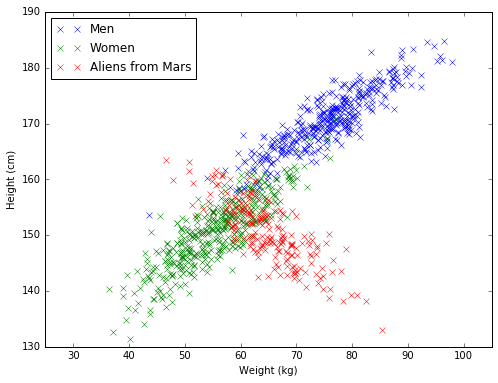# Gaussian Discriminant Analysis¶

(Reference - see chapter 4 of Murphy's MLPP)

In class, you talked about multivariate mixture of gaussian models fowhere we assumed your dataset was generated according to the following generative process: 1) We sample a class from a Categorical Distribution, $$Cat(y|\theta) = \prod_{j=1}^K \theta_j^{\mathbb{I}(y_j=1)}$$ 2) Given the class, the features of a particular obervation were sampled from a multivariate normal with class-specific mean and covariance. $$MVN(x|y = c, \mu_c, \Sigma_c) = (2\pi)^{-D/2}|\Sigma|^{-1/2}\exp\left[-\frac{1}{2}(x-\mu_c)^T\Sigma^{-1}_c(x-\mu_c)\right]$$

Today, we're assuming the same generative process, except the we assume that we have the class labels, $y_i$, and we're doing supervised learning.

As a running example, we assumed we had a dataset of heights and weights of men, women and aliens from Mars (who's weight turns out to be inversely proportional to their height so that our plot looks a little less boring...).

In :
import matplotlib.pyplot as plt
import numpy as np
%matplotlib inline

n = 1000
X = np.zeros((n,2)) # initialize our data
y = np.zeros((n, 3))

true_theta = [0.4, 0.4, 0.2] # class probabilities

#class means
true_means = [
[75, 170], #men
[55, 150], #women
[65, 150]  #aliens
]
#class covariances
true_cov = [[[80, 50], [-5, 2]],
[[60, 50], [-8, 2]],
[[60, -50], [8, 2]]
]

for i in xrange(n):
# note - this code would be a lot faster if you vectorized this loop...

# sample a class label
y[i,:] = np.random.multinomial(1, true_theta, size=1).flatten()
c = np.argmax(y[i,:])
# sample the corresponding features.
X[i,:] = np.random.multivariate_normal(true_means[c], true_cov[c], 1).flatten()

plt.figure(figsize=(8,6))
plt.plot(X[y[:,0]==1,0], X[y[:,0]==1,1], 'x', label='Men')
plt.plot(X[y[:,1]==1,0], X[y[:,1]==1,1], 'x', label='Women')
plt.plot(X[y[:,2]==1,0], X[y[:,2]==1,1], 'x', label='Aliens from Mars')
plt.axis('equal')
plt.xlabel('Weight (kg)')
plt.ylabel('Height (cm)')
plt.legend(loc=2)
plt.show()To fit the parameters of the model, we're going to derive closed-form maximum likelihood estimators (this works out because we're working with Gaussians). For this tutorial, we're going to assume that all of our Gaussians share the same covariance matrix. i.e. $$\Sigma_1 = \Sigma_2 = ... = \Sigma_c = \Sigma$$ (note that this is clearly an inappropriate assumption for the data that we're modeling because our aliens have very different covariance to the men and women - we'll ignore this for now). The resulting model is known as a Linear Discriminant Analysis model (see Murphy - ch. 4).

We can write the log-likelihood function as follows: $$\mathcal{l}(\mathcal{x} | \theta, \mu_i, \Sigma_i) = \sum_{i=1}^n \log p(x_i | y = y_i,\mu_{y_i}, \Sigma_{y_i} ) + \log p(y_i | \theta)$$

## MLE categorical distribution¶

To maximise $\mathcal{l}()$ with respect to $\theta$, we're finding the MLE of a categorical distribution since, $$\nabla_\theta \mathcal{l}(\mathcal{x} | \theta, \mu_i, \Sigma_i) = \sum_{i=1}^n\nabla_\theta \log p(y_i | \theta) =\sum_{i=1}^n \nabla_\theta \log \prod_{j=1}^K \theta_j^{\mathbb{I}(y_j=1)} =\sum_{i=1}^n \sum_{j=1}^K \nabla_\theta \log \theta_j^{\mathbb{I}(y_j=1)}$$

We have to remember to constrain $\theta$ to sum to 1 which we can do by adding the appropriate legrange multiplier. Our Legrangian is as follows: $$L(\theta) = \sum_{i=1}^n \sum_{j=1}^K \nabla_\theta \log \theta_j^{\mathbb{I}(y_j=1)} - \lambda (\sum_l \theta_l - 1)$$

$$\frac{\partial L}{\partial \theta_c} = 0 \Rightarrow \sum_{i=1}^n \mathbb{I}(y_i = c)\frac{1}{\theta_c} = \lambda \Rightarrow n_c = \lambda \theta_c$$

Where $n_c$ is the number of examples where $y_i$ is class $c$.

Now summing over all classes, and using the fact that $\sum_c\theta_c = 1$ and $\sum_c n_c = n$, we get: $$\sum_c n_c = \sum_c\lambda \theta_c \Rightarrow n = \lambda$$ Putting this together, we get that the MLE for $\theta_c$ is, $$\theta_c = \frac{n_c}{n}$$

In our running example, we could calculate this as follows:

In :
theta_hat = y.mean(axis=0)
print theta_hat

[ 0.38   0.418  0.202]


## MLE means¶

Let's now calculate the MLE of $\mu_c$. $$\nabla_{\mu_c} \mathcal{l}(\mathcal{x} | \theta, \mu_i, \Sigma_i) = \nabla_{\mu_c} \sum_{i=1}^n \log p(x_i | y = y_i,\mu_{y_i}, \Sigma_{y_i} )$$ $$= \sum_{i=1}^n \mathbb{I}(y_i = c) \nabla_{\mu_c}\log p(x_i | y = c,\mu_{c}, \Sigma_{c} )$$ $$= \sum_{i=1}^n \mathbb{I}(y_i = c) \nabla_{\mu_c}[\log (2\pi)^{-D/2} + \log |\Sigma|^{-1/2} + \left[-\frac{1}{2}(x_i-\mu_c)^T\Sigma^{-1}_c(x_i-\mu_c)\right] )]$$ $$= \sum_{i=1}^n \mathbb{I}(y_i = c) \nabla_{\mu_c} \left[-\frac{1}{2}(x_i-\mu_c)^T\Sigma^{-1}_c(x_i-\mu_c)\right] )$$ $$= \sum_{i=1}^n \mathbb{I}(y_i = c)\, \Sigma^{-1}_c(x_i-\mu_c)$$ Where the last line follows from the identity $\nabla_s (x-s)^TW(x-s) = -2W(x-s)$ that holds is $W$ is symmetric (see Matrix Cookbook). Setting this equal to zero, we get: $$\sum_{i=1}^n \mathbb{I}(y_i = c)\, x_i = \mu_c \sum_{i=1}^n \mathbb{I}(y_i = c) = \mu_c n_c$$

$$\mu_c = \frac{1}{n_c} \sum_{i=1}^n \mathbb{I}(y_i = c)\, x_i$$

In code, this would be:

In :
n_men = float((y[:,0] == 1).sum())
mu_men = 1/n_men * X[y[:,0] == 1, :].sum(axis=0)

n_women = float((y[:,1] == 1).sum())
mu_women = 1/n_women * X[y[:,1] == 1, :].sum(axis=0)

n_aliens = float((y[:,2] == 1).sum())
mu_aliens = 1/n_aliens * X[y[:,2] == 1, :].sum(axis=0)
print mu_men, mu_women, mu_aliens

[  75.49045953  170.58300886] [  55.44694581  150.67175308] [  64.86236075  150.43012944]


## MLE Covarience¶

$$\nabla_{\Sigma} \mathcal{l}(\mathcal{x} | \theta, \mu_i, \Sigma_i) = \sum_{i=1}^n \nabla_{\Sigma}[\log |\Sigma|^{-1/2} + \left[-\frac{1}{2}(x_i-\mu_{y_i})^T\Sigma^{-1}_c(x_i-\mu_{y_i})\right] )]$$

Using the same trace trick and gradient with respect to the log determinant that we saw in class, we can get: $$\nabla_{\Sigma} \mathcal{l}(\mathcal{x} | \theta, \mu_i, \Sigma_i) = \sum_{i=1}^n \frac{-1}{2}\Sigma +\frac{1}{2}(x_i-\mu_{y_i})(x_i-\mu_{y_i})^T)]$$

Setting this equal to zero, we get: $$\sum_{i=1}^n \Sigma = \sum_{i=1}^n (x_i-\mu_{y_i})(x_i-\mu_{y_i})^T)]$$

$$\Sigma = \frac{1}{n} \sum_{i=1}^n (x_i-\mu_{y_i})(x_i-\mu_{y_i})^T)]$$

I.e. in linear discriminant analysis, we subtract off the class specific mean from each feature and then compute the sample covariance

## Relationship to logistic regression¶

Finally, we noticed that we could reparameterize the model as follows: $$p(y = c|x, \mu_i, \Sigma, \theta) = \frac{p(x|y, \mu_c, \Sigma) * p(y = c|\theta)}{\sum_{c'}p(x|y, \mu_{c'}, \Sigma) * p(y = c'|\theta)}$$

$$= \frac{(2\pi)^{-D/2}|\Sigma|^{-1/2}\exp\left[-\frac{1}{2}(x-\mu_c)^T\Sigma^{-1}(x-\mu_c)\right]* \theta_{c}}{\sum_{c'}(2\pi)^{-D/2}|\Sigma|^{-1/2}\exp\left[-\frac{1}{2}(x-\mu_{c'})^T\Sigma^{-1}(x-\mu_{c'})\right]* \theta_{c'}}$$$$= \frac{\exp\left[-\frac{1}{2}(x-\mu_c)^T\Sigma^{-1}(x-\mu_c) + \log\theta_{c}\right]}{\sum_{c'}\exp\left[-\frac{1}{2}(x-\mu_{c'})^T\Sigma^{-1}(x-\mu_{c'}) + \log\theta_{c'}\right]}$$$$= \frac{\exp\left[-\frac{1}{2}x^T\Sigma^{-1}x +\mu_c^T\Sigma^{-1}x -\frac{1}{2}\mu_c^T\Sigma^{-1}\mu_c + \log\theta_{c}\right]}{\sum_{c'}\exp\left[-\frac{1}{2}x^T\Sigma^{-1}x +\mu_{c'}^T\Sigma^{-1}x -\frac{1}{2}\mu_{c'}^T\Sigma^{-1}\mu_{c'} + \log\theta_{c'}\right]}$$$$= \frac{\exp(-\frac{1}{2}x^T\Sigma^{-1}x)\exp\left[\mu_c^T\Sigma^{-1}x -\frac{1}{2}\mu_c^T\Sigma^{-1}\mu_c + \log\theta_{c}\right]}{\sum_{c'}\exp(-\frac{1}{2}x^T\Sigma^{-1}x)\exp\left[\mu_{c'}^T\Sigma^{-1}x -\frac{1}{2}\mu_{c'}^T\Sigma^{-1}\mu_{c'} + \log\theta_{c'}\right]}$$$$= \frac{\exp\left[\mu_c^T\Sigma^{-1}x -\frac{1}{2}\mu_c^T\Sigma^{-1}\mu_c + \log\theta_{c}\right]}{\sum_{c'}\exp\left[\mu_{c'}^T\Sigma^{-1}x -\frac{1}{2}\mu_{c'}^T\Sigma^{-1}\mu_{c'} + \log\theta_{c'}\right]}$$

If we let $\beta_c = \mu_c^T\Sigma^{-1}$ and $\alpha_c = -\frac{1}{2}\mu_c^T\Sigma^{-1}\mu_c + \log\theta_{c}$, we can re-write this as:

$$= \frac{\exp\left(\beta_c^Tx + \alpha_c\right)}{\sum_{c'} \exp\left(\beta_{c'}^Tx + \alpha_{c'} \right)}$$

Which is exactly the functional form of multi-class logistic regression. So we've built a generative model, but our assumptions on $\Sigma$ have lead to a linear decision boundry that relates to a descriminiative model. See section 8.6 of Murphy if you're interested in reading more about this.

In [ ]: